#### Homeomorphism: Making a donut into a coffee cup

You might have heard the expression that to a topologist, a donut and a coffee cup appear the same. In many branches of mathematics, it is important to define when two basic objects are equivalent. In graph theory (and group theory), this equivalence relation is called an isomorphism. In topology, the most basic equivalence is a homeomorphism, which allows spaces that appear quite different in most other subjects to be declared equivalent in topology. The surfaces of a donut and a coffee cup (with one handle) are considered equivalent because both have a single hole. This notion needs to be made more precise!

Suppose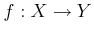is a bijective (one-to-one and onto) function between topological spacesand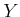. Since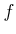is bijective, the inverse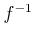exists. If bothandare continuous, thenis called a homeomorphism. Two topological spacesandare said to be homeomorphic, denoted by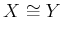, if there exists a homeomorphism between them. This implies an equivalence relation on the set of topological spaces (verify that the reflexive, symmetric, and transitive properties are implied by the homeomorphism).

Example 4..5 (Interval Homeomorphisms)   Any open interval ofis homeomorphic to any other open interval. For example,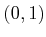can be mapped to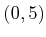by the continuous mapping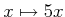. Note thatandare each being interpreted here as topological subspaces of. This kind of homeomorphism can be generalized substantially using linear algebra. If a subset,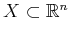, can be mapped to another,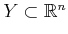, via a nonsingular linear transformation, thenandare homeomorphic. For example, the rigid-body transformations of the previous chapter were examples of homeomorphisms applied to the robot. Thus, the topology of the robot does not change when it is translated or rotated. (In this example, note that the robot itself is the topological space. This will not be the case for the rest of the chapter.)

Be careful when mixing closed and open sets. The space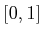is not homeomorphic to, and neither is homeomorphic to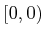. The endpoints cause trouble when trying to make a bijective, continuous function. Surprisingly, a bounded and unbounded set may be homeomorphic. A subsetof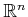is called bounded if there exists a ball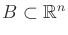such that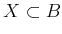. The mappingestablishes thatand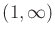are homeomorphic. The mapping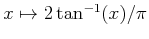establishes that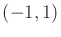and all ofare homeomorphic!Example 4..6 (Topological Graphs)   Letbe a topological space. The previous example can be extended nicely to make homeomorphisms look like graph isomorphisms. Let a topological graph4.2 be a graph for which every vertex corresponds to a point inand every edge corresponds to a continuous, injective (one-to-one) function,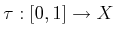. The image of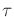connects the points inthat correspond to the endpoints (vertices) of the edge. The images of different edge functions are not allowed to intersect, except at vertices. Recall from graph theory that two graphs,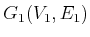and, are called isomorphic if there exists a bijective mapping,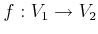such that there is an edge between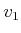and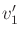in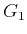, if and only if there exists an edge between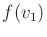and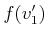in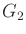.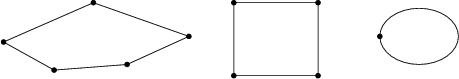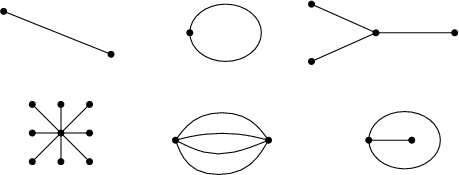The bijective mapping used in the graph isomorphism can be extended to produce a homeomorphism. Each edge in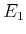is mapped continuously to its corresponding edge in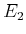. The mappings nicely coincide at the vertices. Now you should see that two topological graphs are homeomorphic if they are isomorphic under the standard definition from graph theory.4.3 What if the graphs are not isomorphic? There is still a chance that the topological graphs may be homeomorphic, as shown in Figure 4.2. The problem is that there appear to be useless'' vertices in the graph. By removing vertices of degree two that can be deleted without affecting the connectivity of the graph, the problem is fixed. In this case, graphs that are not isomorphic produce topological graphs that are not homeomorphic. This allows many distinct, interesting topological spaces to be constructed. A few are shown in Figure 4.3.Steven M LaValle 2020-08-14